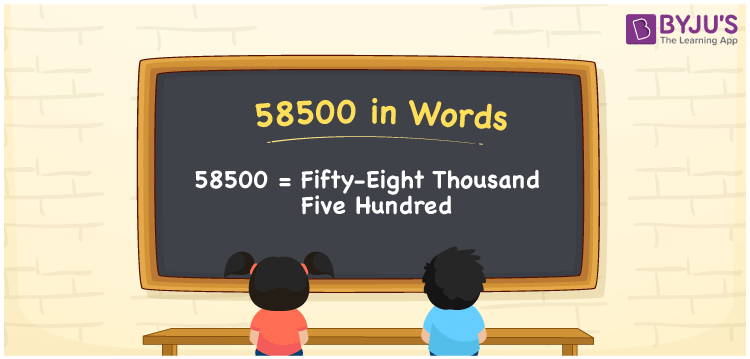# 58500 in Words

58500 in words is Fifty-eight Thousand Five Hundred. For example, if you earned Rs. 58500 in a month, you can write, “I have earned Rs. Fifty-eight Thousand Five Hundred in a month”. In general, the number name of any number can be written using the ones, tens, hundreds, and thousands place of a number. Thus, the place value chart is useful for writing the number 58500 in words.

 58500 in words Fifty-eight Thousand Five Hundred Fifty-eight Thousand Five Hundred in Numbers 58500

## 58500 in English Words## How to Write 58500 in Words?

We can convert 58500 to words using a place value chart. This can be done as follows. The number 58500 has 5 digits, so let’s make a chart that shows the place value up to 5 digits.

 Ten thousands Thousands Hundreds Tens Ones 5 8 5 0 0

Thus, we can write the expanded form as:

5 × Ten thousand + 8 × Thousand + 5 × Hundred + 0 × Ten + 0 × One

= 5 × 10000 + 8 × 1000 + 5 × 500 + 0 × 10 + 0 × 1

= 58500

= Fifty-eight Thousand Five Hundred

58500 is the natural number that is succeeded by 58499 and preceded by 58501.

58500 in words – Fifty-eight Thousand Five Hundred

Is 58500 an odd number? – No.

Is 58500 an even number? – Yes

Is 58500 a perfect square number? – No

Is 58500 a perfect cube number? – No

Is 58500 a prime number? – No

Is 58500 a composite number? – Yes

## Frequently Asked Questions on 58500 in Words

Q1

### How to write 58500 in words?

58500 in English is written as “Fifty-eight Thousand Five Hundred”.
Q2

### Is the number 58500 an odd number?

No, the number 58500 is not an odd number.
Q3

### Is 58500 a composite number?

Yes, 58500 is a composite number.